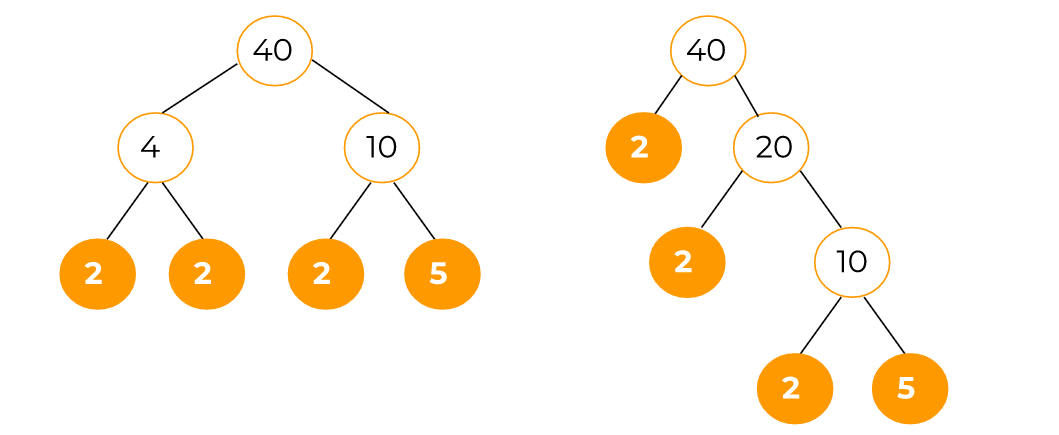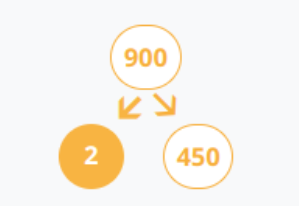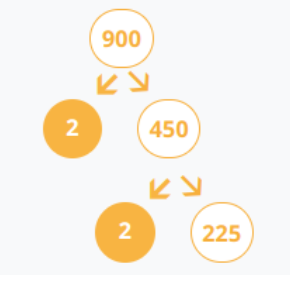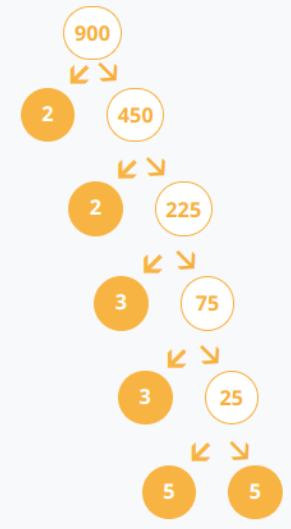# Prime Factor Calculator

To find prime factors, enter number, and click calculate button using prime factor calculator## Prime Factor Calculator

Find the prime factors of any number using the prime factor calculator. Also, see the factor tree for the entered number.

## What are prime factors?

There are two words; prime and factors.

Prime numbers can be divided by only 1, besides themselves. Factors are the numbers that contain the number in question in their multiplication table e.g 1, 2, 3, and 6 are factors of 6.

Now the prime factors make a set of factors that consists only of prime numbers.

### Prime factorization:

The process of breaking a number into factors that are only prime numbers is called prime factorization.

## Methods of prime factorization:

Two famous methods are:

1. Test and trial.
2. Factor tree/ prime decomposition

In the first method, you simply test each prime number starting from 2 until no more decomposition is possible. It can get stressful for bigger numbers.

## How to do prime factorization using the factor tree?

This is the method used in the tool above. The number is divided by the likeliest number that comes to mind. After that, if possible the new two numbers are again divided. This process goes on until all that is left are prime numbers.Both give the same result.

It takes a little less time than the test and trial method because you don’t have to brainstorm. Nonetheless, prime factorization is tedious.

Lastly, prime factors of number can be written in two ways; comma-separated values and exponential form.

Example:

Perform prime factorization for 900.

Solution:

Step 1: Divide 900 by any possible factor.

“2” comes to mind instantly. But you can divide by 300 as well or any other number you seem fitting.Step 2: 2 is a prime number so divide 450 now.

Again 2 can do the job.If you had divided with some other number let's say 60, then divide 60 with its factors as well until it is decomposed to prime numbers.

That is why it is better to start with the smallest numbers.

Step 3: Keep dividing until fully solved.Step 4: List all the prime numbers.

All the colored bubbles are prime numbers.

900 = 2 x 2 x 3 x 3 x 5 x 5

In CSV form:
900 = 2, 2, 3, 3, 5, 5

In exponential form:
900 = 22, 32, 52